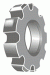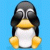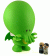•http://facebook.com/
•https://www.google.com/accounts/o8/id
•https://me.yahoo.com

# Graphical Equations for Excel (alpha)1 Jul 08, 8:23PM
(10 replies)
Graphical Equations for Excel (alpha)
Here is our first attempt to produce nice LaTeX equations directly within Excel. What we're doing is converting an Excel formula, i.e.
=sin(1+2/3)
into LaTeX, which is then rendered graphically and displayed next to your equation. All within Excel.

There are two uses for this. First its a handy way to checking that the equation you've typed on a single line is precisely what you want; second graphical equations are a much nicer way to display the equations you've used, so others can read your sheets more easily.

To use this system, you simply need the following Macro:
Sub LatexIT()
Dim equation As Variant
equation = Selection.Formula
If (equation <> "") Then
ActiveCell.Offset(0, 1).Select
ActiveSheet.Pictures.Insert("http://www.codecogs.com/excel.latex?" + equation).Select
With Selection.ShapeRange
.Fill.Solid
.Fill.Transparency = 0#
.Top = ActiveCell.Top - (.Height - ActiveCell.Height) / 2
.IncrementLeft 5
End With
End If
End Sub

So within Excel, goto menu Tools->Macro, type in the name you want for the macro (we've used LatexIT), click create and paste in the code above. You'll probably also want to assign it to a key sequence, i.e. Ctrl+e.

To see one we did earlier, download this: Now back in your excel sheet, type in a formula, then run the macro (i.e. Ctrl e), and if you've done everything right the equation will appear to the right. You can then move this to where-ever you want.

This is only the alpha, we're working through a variety of different Excel formula forms to get them all to display nicely. We'll keep developing the system and report any major improvement here. Please let us know your thoughts.2 Jul 08, 1:11PM
Doesn't currently work with Excel 2007.2 Jul 08, 5:40PM
There are a ton of similar forum posts with people observing that Excel 2007 doesn't work as it should. Furthermore when you record a macro, Excel 2007 doesn't insert the code when it comes to shapes. So we're in the dark here.

I've come across this code which solves one problem in 2007, i.e. putting all images in cell B2:
Sub LatexIT2007()
Dim equation As Variant
equation = Selection.Formula
With ActiveSheet.Pictures.Insert("http://www.codecogs.com/excel.latex?" + equation)
.Left = ActiveCell.Offset(0, 1).Left
.Top = ActiveCell.Offset(0, 1).Top
End With
End Sub

This isn't a fix, since it appear Excel is unhappy with my url. However I'm just hoping others will see this and can help find a solution.2 Jul 08, 10:23PM
This is great! I would definitely use it, but the one little thing I don't like is when the formula contains cell references (eg. 'D1') the references appear in the graphic and spoil it a bit. This is not really your fault though.

Fortunately if you name a cell in Excel (using the Name Box just to the left of the formula bar) and then refer to the cell using name instead of reference, and that looks a lot better (more like a proper equation). But I wonder how many Excel users even know about the Name Box?

What could be nice is if your macro could check all cell references in the formula to see if the cells had already been named - and then update the formula to use the names instead - else prompt the user for a name (optional) for each reference which would then also be saved (possibly via the Name Box).3 Jul 08, 10:01AM
Like the idea of checking to see if an equation already has a name assigned previously. Generally Excel does this automatically if you click on a cell that is named, but it wouldn't if you had typed it in by hand.

I guess the question is how far do we go. If we start modifying equations from the form they were originally, then we run the danger of producing an equation that differs from what the user really wanted. i.e. could there be circumstances when they really do what the references not the name reference?3 Jul 08, 10:03AM
Thanks for all the great feedback.

Bug with multiplying two cell references, i.e.
=A1*D3
has been fixed and should display correctly, i.e.3 Jul 08, 10:44AM
Just added a small modification to color cell references in blue, i.e the formula
=A3*2/sqrt(6*D7)
becomes3 Jul 08, 12:03PM
A new version of the Macro that puts a box around the equation
Sub LatexITBox()
Dim equation As Variant
equation = Selection.Formula
If (equation <> "") Then
ActiveCell.Offset(0, 1).Select
ActiveSheet.Pictures.Insert("http://www.codecogs.com/excel.latex?" + equation).Select
With Selection.ShapeRange
.Fill.Solid
.Fill.Transparency = 0#
.Top = ActiveCell.Top - (.Height - ActiveCell.Height) / 2
.IncrementLeft 5

.PictureFormat.CropLeft = -2.83
.PictureFormat.CropRight = -2.83
.PictureFormat.CropTop = -2.83
.PictureFormat.CropBottom = -2.83
.Line.Visible = msoTrue
.Line.ForeColor.SchemeColor = 23
End With
End If
End Sub4 Jul 08, 10:21PM
Ok, so maybe changing the user's formulae for them isn't such a good idea. You have gone for a good compromise by colouring the cell references - they don't spoil the equation anymore now. Nice touch with the box too!5 Jul 08, 11:11AM
Thanks. Another style issue I'd like thoughts on. What do we do with functions names, i.e
=IF(A1>10,"Big","Little")
I'm converting this into

Notice, I'm converting all uppercase excel function names to lower case. Now with functions like SIN, COS, TAN etc, they are more usually written in maths notation in lower case. However should I make everything lower case, or everything uppercase.

Perhaps I should write functions in a different color also - green perhaps, i.e.5 Jul 08, 8:38PM
I would colour the function names in, like you have done, but perhaps it would be a good idea to have 2 colours: 1 for user-defined functions and 1 for Excel's functions.

The case question is a bit of tough one. Initially I thought that you should leave the case unchanged, so people could tell when an Excel function is being used. But for functions like SIN, do you really need to know this? And what if you wanted to use capitals yourself, let us not go starting conventions that we're going to immediately break!

The problem with changing the case is that useful information can be lost - if camel case or acronyms are used - so case SHOULD be preserved...

With one exception... Excel functions which are only uppercase should become lowercase, like you are already doing... With one proviso - use 2 colours, so Excel functions can be distinguished.